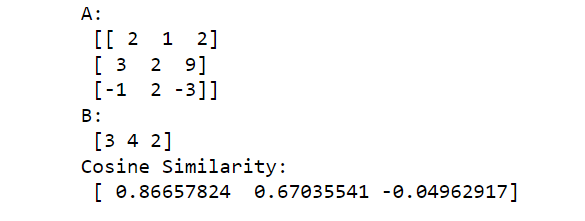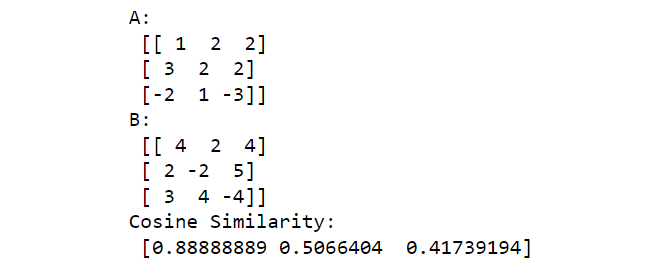# How to Calculate Cosine Similarity in Python?

• Last Updated : 14 Mar, 2022

In this article, we calculate the Cosine Similarity between the two non-zero vectors. A vector is a single dimesingle-dimensional signal NumPy array. Cosine similarity is a measure of similarity, often used to measure document similarity in text analysis. We use the below formula to compute the cosine similarity.

`Similarity = (A.B) / (||A||.||B||) `

where A and B are vectors:

• A.B is dot product of A and B: It is computed as sum of element-wise product of A and B.
• ||A|| is L2 norm of A: It is computed as square root of the sum of squares of elements of the vector A.

Example 1:

In the example below we compute the cosine similarity between the two vectors (1-d NumPy arrays).  To define a vector here we can also use the Python Lists.

## Python

 `# import required libraries``import` `numpy as np``from` `numpy.linalg ``import` `norm` `# define two lists or array``A ``=` `np.array([``2``,``1``,``2``,``3``,``2``,``9``])``B ``=` `np.array([``3``,``4``,``2``,``4``,``5``,``5``])` `print``(``"A:"``, A)``print``(``"B:"``, B)` `# compute cosine similarity``cosine ``=` `np.dot(A,B)``/``(norm(A)``*``norm(B))``print``(``"Cosine Similarity:"``, cosine)`

Output:Example 2:

In the below example we compute the cosine similarity between a batch of three vectors (2D NumPy array) and a vector(1-D NumPy array).

## Python

 `# import required libraries``import` `numpy as np``from` `numpy.linalg ``import` `norm` `# define two lists or array``A ``=` `np.array([[``2``,``1``,``2``],[``3``,``2``,``9``], [``-``1``,``2``,``-``3``]])``B ``=` `np.array([``3``,``4``,``2``])``print``(``"A:\n"``, A)``print``(``"B:\n"``, B)` `# compute cosine similarity``cosine ``=` `np.dot(A,B)``/``(norm(A, axis``=``1``)``*``norm(B))``print``(``"Cosine Similarity:\n"``, cosine)`

Output:

Notice that A has three vectors and B is a single vector. In the above output, we get three elements in the cosine similarity array. The first element corresponds to the cosine similarity between the first vector (first row) of A and the second vector (B). The second element corresponds to the cosine similarity between the second vector (second row ) of A and the second vector (B). And similarly for the third element.Example 3:

In the below example we compute the cosine similarity between the two 2-d arrays. Here each array has three vectors. Here to compute the dot product using the m of element-wise product.

## Python

 `# import required libraries``import` `numpy as np``from` `numpy.linalg ``import` `norm` `# define two arrays``A ``=` `np.array([[``1``,``2``,``2``],``               ``[``3``,``2``,``2``],``               ``[``-``2``,``1``,``-``3``]])``B ``=` `np.array([[``4``,``2``,``4``],``               ``[``2``,``-``2``,``5``],``               ``[``3``,``4``,``-``4``]])` `print``(``"A:\n"``, A)``print``(``"B:\n"``, B)` `# compute cosine similarity``cosine ``=` `np.``sum``(A``*``B, axis``=``1``)``/``(norm(A, axis``=``1``)``*``norm(B, axis``=``1``))` `print``(``"Cosine Similarity:\n"``, cosine)``print``(``"Cosine Similarity:\n"``, cosine)`

Output:

The first element of the cosine similarity array is a similarity between the first rows of A and B. Similarly second element is the cosine similarity between the second rows of A and B. Similarly for the third element.My Personal Notes arrow_drop_up